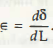What is a strain? The strain is a unit elongation. If there is a bar L and we suspended a load P which is axial, then the elongation takes place only due to the suspended load P. This may be a unit elongation. It is considered as dimensionless. This is also known as longitudinal strain and for that the expression can be written asThe exact knowledge of strain can easily be provided through the following diagram –Here, you just need to understand the factor unit elongation, because if the load is small only then the strain produced a small distance, which is the exact resultant strain. Here, δ is the exact strain due to the loaded force P. In addition c Is also considered as the average strain of the provided object. There are some conditions where strain can be equal to the stress. So, to understand each factor fundamentals are important to clear first.

Links of Next Mechanical Engineering Topics:-### Customer Reviews

My Homework Help
Rated 5.0 out of 5 based on 510 customer reviews at